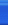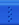# 实时阴影绘制技术研究C++博客首页新随笔联系聚合管理• Pass 2:
1. 不计算光照，利用VS绘制从视点看过去的场景z值深度，输出一个POSITION和一个TEXTURE0，其中TEXTURE0保存了从光源到该点的深度（使用了纹理矩阵来处理从视点可见的点即可得到），此纹理输出给PS使用。
3. 该pass的颜色输出到纹理g_pSceneSurf,深度模板表面保存在g_pNewDetphSurf中。
• Pass 3：
2. //
// Gaussian functions(高斯函数)
//
float GetGaussianDistribution( float x, float y, float rho ) {
float g = 1.0f / sqrt( 2.0f * 3.141592654f * rho * rho );
return g * exp( -(x * x + y * y) / (2 * rho * rho) );
}

void GetGaussianOffsets( bool bHorizontal, D3DXVECTOR2 vViewportTexelSize,
D3DXVECTOR2* vSampleOffsets, float* fSampleWeights ) {
// Get the center texel offset and weight
fSampleWeights = 1.0f * GetGaussianDistribution( 0, 0, 2.0f );
vSampleOffsets = D3DXVECTOR2( 0.0f, 0.0f );

// Get the offsets and weights for the remaining taps
if( bHorizontal ) {
for( int i = 1; i < 15; i += 2 ) {
vSampleOffsets[i + 0] = D3DXVECTOR2(  i * vViewportTexelSize.x, 0.0f );
vSampleOffsets[i + 1] = D3DXVECTOR2( -i * vViewportTexelSize.x, 0.0f );

fSampleWeights[i + 0] = 2.0f * GetGaussianDistribution( float(i + 0), 0.0f, 3.0f );
fSampleWeights[i + 1] = 2.0f * GetGaussianDistribution( float(i + 1), 0.0f, 3.0f );
}
}

else {
for( int i = 1; i < 15; i += 2 ) {
vSampleOffsets[i + 0] = D3DXVECTOR2( 0.0f,  i * vViewportTexelSize.y );
vSampleOffsets[i + 1] = D3DXVECTOR2( 0.0f, -i * vViewportTexelSize.y );

fSampleWeights[i + 0] = 2.0f * GetGaussianDistribution( 0.0f, float(i + 0), 3.0f );
fSampleWeights[i + 1] = 2.0f * GetGaussianDistribution( 0.0f, float(i + 1), 3.0f );
}
}
}
具体的数学公式我没有查，大概看不懂，还望高手指点。
• Pass4：这二遍做垂直模糊。